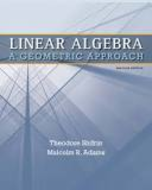### Solution Found!# The equation 2x1 3x2 6x3 = 4 defines a plane in R3. a. Give its normal vector a. b. Find

Chapter 1, Problem 8

(choose chapter or problem)

Get Unlimited Answers! Check out our subscriptions
QUESTION:

The equation $$2 x_{1}-3 x_{2}-6 x_{3}=-4$$ defines a plane in $$\mathbb{R}^{3}$$.

a. Give its normal vector a.

b. Find the distance from the origin to the plane by using projection.

c. Find the point on the plane closest to the origin by using the parametric equation of the line through $$0$$ with direction vector a. Double-check your answer to part b.

d. Find the distance from the point $$\mathbf{w}=(3,-3,-5)$$ to the plane by using projection.

e. Find the point on the plane closest to w by using the parametric equation of the line through w with direction vector a. Double-check your answer to part d.

### Questions & Answers (3 Reviews)

QUESTION:

The equation $$2 x_{1}-3 x_{2}-6 x_{3}=-4$$ defines a plane in $$\mathbb{R}^{3}$$.

a. Give its normal vector a.

b. Find the distance from the origin to the plane by using projection.

c. Find the point on the plane closest to the origin by using the parametric equation of the line through $$0$$ with direction vector a. Double-check your answer to part b.

d. Find the distance from the point $$\mathbf{w}=(3,-3,-5)$$ to the plane by using projection.

e. Find the point on the plane closest to w by using the parametric equation of the line through w with direction vector a. Double-check your answer to part d.

Step 1 of 9

Given plane: $$2{x_1} - 3{x_2} - 6{x_3} = - 4$$ in $$\text{R}^3$$.

### Review this written solution for 964057) viewed: 340 isbn: 9781429215213 | Linear Algebra: A Geometric Approach - 2 Edition - Chapter 1.3 - Problem 8

Thank you for your recent purchase on StudySoup. We invite you to provide a review below, and help us create a better product.

Textbook: Linear Algebra: A Geometric Approach

Click to rate

Write a review below (optional):

### Review this written solution for 964057) viewed: 340 isbn: 9781429215213 | Linear Algebra: A Geometric Approach - 2 Edition - Chapter 1.3 - Problem 8

Thank you for your recent purchase on StudySoup. We invite you to provide a review below, and help us create a better product.

Textbook: Linear Algebra: A Geometric Approach

Click to rate

Write a review below (optional):

### Review this written solution for 964057) viewed: 340 isbn: 9781429215213 | Linear Algebra: A Geometric Approach - 2 Edition - Chapter 1.3 - Problem 8

Thank you for your recent purchase on StudySoup. We invite you to provide a review below, and help us create a better product.

Textbook: Linear Algebra: A Geometric Approach

Click to rate

Write a review below (optional):